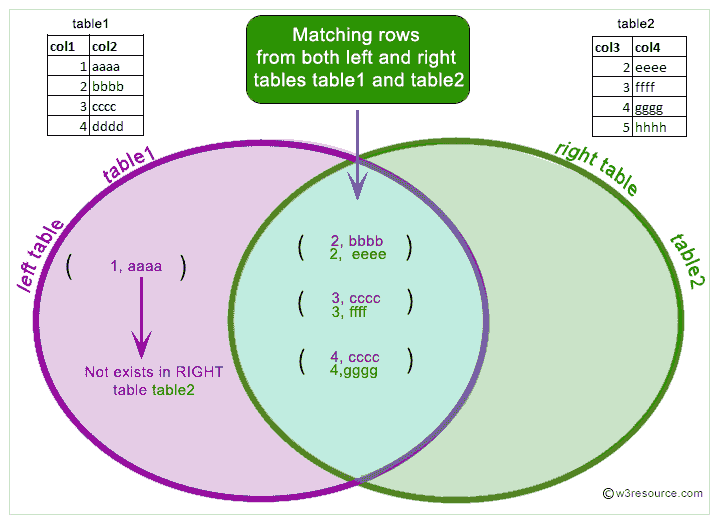# MySQL LEFT JOIN

## What is LEFT JOIN in MySQL?

The MySQL LEFT JOIN joins two tables and fetches rows based on a condition, which is matching in both the tables and the unmatched rows will also be available from the table written before the JOIN clause.

Pictorial presentation of MySQL LEFT JOIN:MySQL LEFT JOIN Syntax :

MySQL supports the following JOIN syntaxes for the table_references (A table reference is also known as a join expression.) part of SELECT statements and multiple-table UPDATE and DELETE statements:

```table_references:
escaped_table_reference [, escaped_table_reference] ...

escaped_table_reference:
table_reference
| { OJ table_reference }

table_reference:
table_factor
| join_table

table_factor:
tbl_name [PARTITION (partition_names)]
[[AS] alias] [index_hint_list]
| table_subquery [AS] alias
| ( table_references )

join_table:
table_reference [INNER | CROSS] JOIN table_factor [join_condition]
| table_reference STRAIGHT_JOIN table_factor
| table_reference STRAIGHT_JOIN table_factor ON conditional_expr
| table_reference {LEFT|RIGHT} [OUTER] JOIN table_reference join_condition
| table_reference NATURAL [{LEFT|RIGHT} [OUTER]] JOIN table_factor

join_condition:
ON conditional_expr
| USING (column_list)

index_hint_list:
index_hint [, index_hint] ...

index_hint:
USE {INDEX|KEY}
[FOR {JOIN|ORDER BY|GROUP BY}] ([index_list])
| IGNORE {INDEX|KEY}
[FOR {JOIN|ORDER BY|GROUP BY}] (index_list)
| FORCE {INDEX|KEY}
[FOR {JOIN|ORDER BY|GROUP BY}] (index_list)

index_list:
index_name [, index_name] ...```

Suppose: 1st_table LEFT JOIN 2nd_table JOIN CONDITION

In MySQL LEFT JOIN, table 2nd_table depends on table 1st_table and all tables on which 1st_table depends and also table 1st_table depends on all tables that are used in the LEFT JOIN condition except 2nd_table. The LEFT JOIN condition is used to decide how to retrieve rows from table 2nd_table.

If there is a row in 1st_table that matches the WHERE clause, but there is no row in 2nd_table that matches the ON condition, an extra 2nd_table row is generated with all columns set to NULL.

So, in case of LEFT JOIN or LEFT OUTER JOIN, MySQL -

1. takes all selected values from the left table

2. combines them with the column names ( specified in the condition ) from the right table

3. retrieve the matching rows from both the associated tables.

4. sets the value of every column from the right table to NULL which is unmatched with the left table.

Example: MySQL LEFT JOIN

A LEFT JOIN will preserve the records of the "left" table. MySQL starts with the left table. For each row from the alias bk1 MySQL scans the table, refers if the condition is satisfied and returns the book name. For unmatched row it returns null. Each item in the left table will be shown in a MySQL result, even if there isn't a match in the other table.

In this example book_mast table is aliased as bk1 and bk2. The INNER JOIN performed in the statement checks whether book_price in bk1 is less than book_price in bk2 and whether the language of the book in bk2 is German; if so, then the statement returns book_name, isbn_no, book_price, pub_lang from bk1.

Notice that this example creates two virtual tables out of book_mast and performs a JOIN between them.

Code:

``````SELECT bk1.book_name,bk1.isbn_no,bk1.book_price,bk1.pub_lang
FROM  book_mast bk1
LEFT JOIN book_mast bk2 ON bk1.book_price<bk2.book_price
WHERE bk2.pub_lang='German';
```
```

Sample table: book_mast :

Sample Output:

```mysql> SELECT bk1.book_name,bk1.isbn_no,bk1.book_price,bk1.pub_lang
-> FROM  book_mast bk1
-> LEFT JOIN book_mast bk2 ON bk1.book_price<bk2.book_price
-> WHERE bk2.pub_lang='German';
+-------------------------------------+-------------+------------+----------+
| book_name                           | isbn_no     | book_price | pub_lang |
+-------------------------------------+-------------+------------+----------+
| Introduction to Electrodynamics     | 0000979001  |      85.00 | English  |
| Understanding of Steel Construction | 0000979002  |     105.50 | English  |
| Advanced 3d Graphics                | 0000979007  |      56.00 | Hindi    |
| Human Anatomy                       | 0000979008  |      50.50 | German   |
| The Experimental Analysis of Cat    | 0000979011  |      95.00 | French   |
| The Nature  of World                | 0000979012  |      88.00 | English  |
| Environment a Sustainable Future    | 0000979013  |     100.00 | German   |
| Networks and Telecommunications     | 00009790_16 |      45.00 | French   |
| Networks and Telecommunications     | 00009790_16 |      45.00 | French   |
| Introduction to Electrodynamics     | 0000979001  |      85.00 | English  |
| Advanced 3d Graphics                | 0000979007  |      56.00 | Hindi    |
| Human Anatomy                       | 0000979008  |      50.50 | German   |
| The Experimental Analysis of Cat    | 0000979011  |      95.00 | French   |
| The Nature  of World                | 0000979012  |      88.00 | English  |
| Networks and Telecommunications     | 00009790_16 |      45.00 | French   |
+-------------------------------------+-------------+------------+----------+
15 rows in set (0.00 sec)
```

PHP script:

``````<!doctype html>
<html lang="en">
<meta charset="utf-8">
<meta name="viewport" content="width=device-width, initial-scale=1">
<title>example-left-join php mysql examples | w3resource</title>
<meta name="description" content="example-left-join php mysql examples | w3resource">
<body>
<div class="container">
<div class="row">
<div class="col-md-12">
<h2>The most costly book in book_mast table is:</h2>
<table class='table table-bordered'>
<tr>
<th>Name of the book</th><th>ISBN no</th><th>Price of the book</th><th>Language of the book</th>
</tr>
<?php
\$hostname="your_hostname";
\$db = "your_dbname";
foreach(\$dbh->query('SELECT bk1.book_name,bk1.isbn_no,bk1.book_price,bk1.pub_lang
FROM  book_mast bk1
LEFT JOIN book_mast bk2 ON bk1.book_price<bk2.book_price
WHERE bk2.pub_lang="German"') as \$row) {
echo "<tr>";
echo "<td>" . \$row['book_name'] . "</td>";
echo "<td>" . \$row['isbn_no'] . "</td>";
echo "<td>" . \$row['book_price'] . "</td>";
echo "<td>" . \$row['pub_lang'] . "</td>";
echo "</tr>";
}
?>
</tbody></table>
</div>
</div>
</div>
</body>
</html>
```
```

View the example in browser

Online Practice Editor:

Key points to remember

Click on the following to get the slides presentation -LEFT JOIN: SQL and other Relational Databases

Previous: INNER JOIN
Next: RIGHT JOIN

﻿